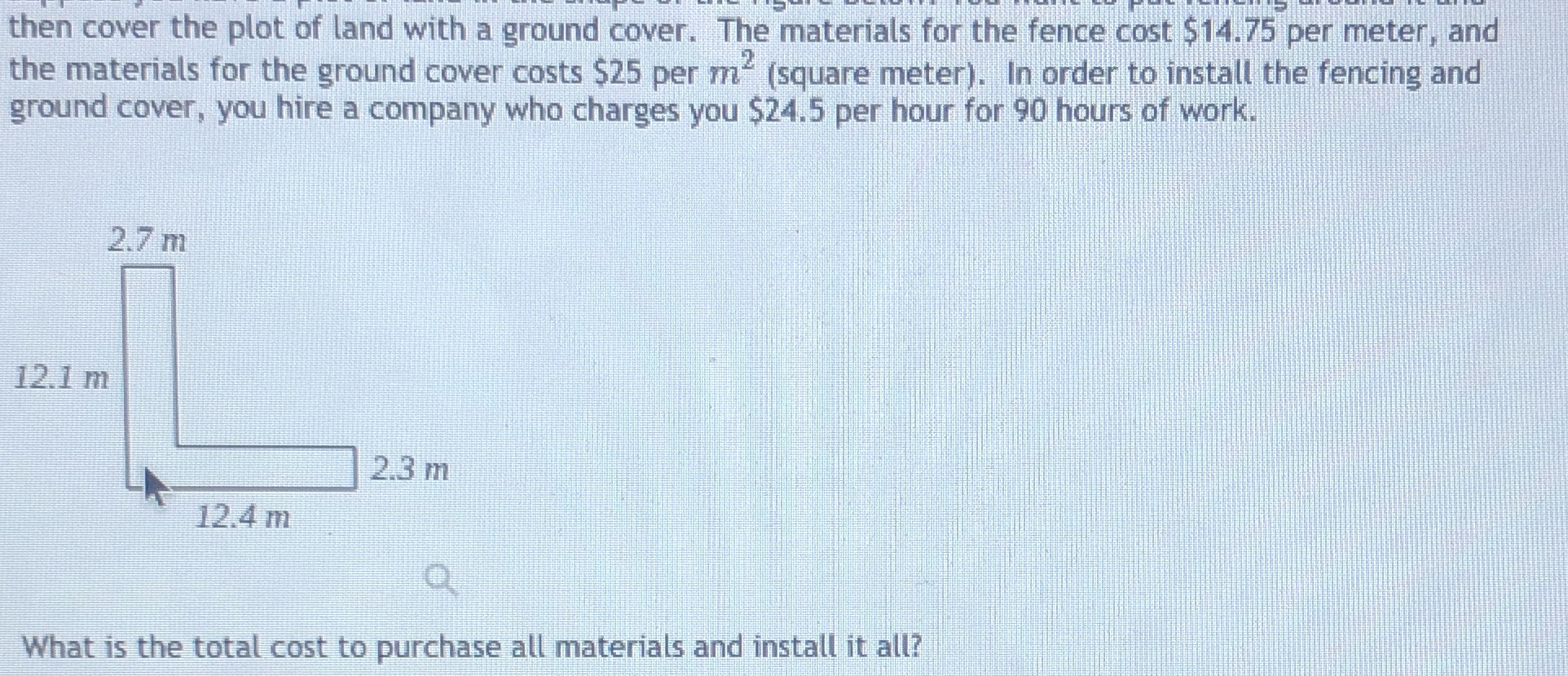### Still have math questions?

Geometry
QuestionThe materials for the fence,cost $$\.75$$ per meter,and the materials for the ground cover costs $$\$$ per $$m^{2}$$(square meter). In order to install the fencing and ground cover,you hire a company who changes you $$\24.5$$ per hour for 90 hours of work.

What is the cost to purchase all materials and install it all?

$$\4302.25$$